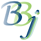# BBjDrawPanel::getFillType

## Description

In BBj 16.0 and higher, this method returns the current fill type for closed objects on the BBjDrawPanel.

## Syntax

 Return Value Method int getFillType()

None.

## Return Value

Returns the current fill type, from the following list:

• 0 = No fill (default)
• 1 = Solid fill
• 2 = Horizontal lines
• 3 = Vertical lines
• 4 = Forward diagonal lines (/)
• 5 = Backward diagonal lines (\)
• 6 = Horizontal and vertical cross-hatch
• 7 = Diagonal cross-hatch

None.

## Example

 ``````rem ' BBjDrawPanel::getFillType sysgui = unt open (sysgui)"X0" sysgui! = bbjapi().getSysGui() title\$ = "BBjDrawPanel::getFillType" window! = sysgui!.addWindow(25,25,1200,750,title\$,\$00090003\$) window!.setCallback(window!.ON_CLOSE,"eoj") window!.setTrack(0) window!.setFont(sysgui!.makeFont("Arial",8,0)) drawpanel! = window!.getDrawPanel() drawpanel!.setPenColor(BBjColor.BLUE) drawpanel!.setFillColor(BBjColor.GREEN) drawpanel!.setTextColor(BBjColor.BLUE) drawpanel!.setRound(5,5) drawpanel!.drawText(10,25,"Shape") drawpanel!.drawText(10,55,"drawPoint") drawpanel!.drawText(10,100,"drawRect") drawpanel!.drawText(10,175,"drawArc") drawpanel!.drawText(10,225,"drawArc") drawpanel!.drawText(10,325,"drawEllipse") drawpanel!.drawText(10,400,"drawArrow") drawpanel!.drawText(10,450,"drawArrow") drawpanel!.drawText(10,500,"drawLine") drawpanel!.drawText(10,575,"drawPolygon") drawpanel!.drawText(10,675,"drawPolyline") print "BBjDrawPanel::getFillType =",drawpanel!.getFillType() for i = 0 to 10 x = i * 100 + 25 drawpanel!.setFillType(i) drawpanel!.setPattern(i) drawpanel!.setPenWidth((i+1)*0.25) drawpanel!.drawPoint(x+100,50) drawpanel!.drawText(x+85,25,"setFillType("+str(i)+")") drawpanel!.drawRect(x+75,75,75,50) r1 = i * Math.PI / 2 r2 = r1 + Math.PI / 2 drawpanel!.drawArc(x+100,175,25,1,r1,r2,1) drawpanel!.drawArc(x+100,225,25,1,r1,r2,0) drawpanel!.drawEllipse(x+75,275,75,50) adj = (i-5) * 5 drawpanel!.moveTo(x+75,400) drawpanel!.drawArrow(x+150,400+adj,0,1) drawpanel!.moveTo(x+75,450) drawpanel!.drawArrow(x+150,450+adj,1,0) drawpanel!.drawLine(x+75,500,x+150,525) points = i + 3 list! = bbjapi().makeVector() for point = 0 to points-1 angle = point * 2 * Math.PI / points px = int(40 * cos(angle) + 110 + x) py = int(40 * sin(angle) + 600) list!.add(px) list!.add(py) next point drawpanel!.drawPolygon(list!) list! = bbjapi().makeVector() for point = 0 to points-1 angle = point * 2 * Math.PI / points px = int(40 * cos(angle) + 110 + x) py = int(40 * sin(angle) + 700) list!.add(px) list!.add(py) next point drawpanel!.drawPolyline(list!) print "BBjDrawPanel::getFillType =",drawpanel!.getFillType() next i process_events eoj: release``````